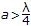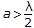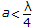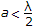# Electronics and Communication Engineering - Microwave Communication

### Exercise :: Microwave Communication - Section 4

31.

Which of the following modes of transmission will not be supported by a rectangular waveguide?

 A. TE15 B. TE12 C. TM11 D. TM10

Explanation:

No answer description available for this question. Let us discuss.

32.

In a circular waveguide with radius r, the dominant mode is

 A. TM01 B. TE01 C. TM11 D. TE11

Explanation:

Some applications require dual polarization capability. Circular waveguide has this capability.

These analysis uses cylindrical coordinates.

In circular waveguide TE11 mode has the lowest cut off frequency and is the dominant mode.

If D is diameter of waveguide

λc = 1.706 D for TE11 mode

λc = 1.029 D for TE21 mode

λc = 0.82 D for TE01 mode

λc = 1.306 D for TM01 mode.

33.

If 'a' is the width of rectangular wave guide and 'λ' is the wave length, then

 A.B.C.D.Explanation:

No answer description available for this question. Let us discuss.

34.

A parametric amplifier uses

 A. non linear resistance B. non linear reactance C. either (a) or (b) D. neither (a) nor (b)

Explanation:

In a parametric amplifier the non-linear element is varactor diode or inductor.

35.

Assertion (A): Impatt diode can be used in both amplifiers and oscillators.

Reason (R): Impatt diode has a low resistance.

 A. Both A and R are correct and R is correct explanation of A B. Both A and R are correct but R is not correct explanation of A C. A is correct but R is wrong D. A is wrong but R is correct

Explanation:

An Impatt diode has n+ - p - i - p + structure and is used with reverse bias.

It exhibits negative resistance and operates on the principle of avalanche breakdown.

Impatt diode circuits are classified as broadly tunable circuit, low Q circuit and high Q circuit.

The impedance of Impatt diode is a few ohms. The word Impatt stands for Impact Avalanche Transit Time diode.

The features of Impatt diode oscillator are : frequency 1 to 300 GHz, Power output (0.5 W to 5 W for single diode circuit and upto 40 W for combination of several diodes), efficiency about 20%.

Its applications include police radar systems, low power microwave transmitter etc.